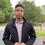# Help with proof!

1) Prove that for any \triangle ABC, we have $$\sin{A}+\sin{B}+\sin{C}\leq \frac{3\sqrt{3}}{2}.$$

2) Let a,b,c be positive real numbers. Prove that

$\frac{a}{\sqrt{a^{2}+8bc}}+\frac{b}{\sqrt{b^{2}+8ca}}+\frac{c}{\sqrt{c^{2}+8ab}}\ge 1$Note by Mardokay Mosazghi
7 years, 2 months ago

This discussion board is a place to discuss our Daily Challenges and the math and science related to those challenges. Explanations are more than just a solution — they should explain the steps and thinking strategies that you used to obtain the solution. Comments should further the discussion of math and science.

When posting on Brilliant:

• Use the emojis to react to an explanation, whether you're congratulating a job well done , or just really confused .
• Ask specific questions about the challenge or the steps in somebody's explanation. Well-posed questions can add a lot to the discussion, but posting "I don't understand!" doesn't help anyone.
• Try to contribute something new to the discussion, whether it is an extension, generalization or other idea related to the challenge.

MarkdownAppears as
*italics* or _italics_ italics
**bold** or __bold__ bold
- bulleted- list
• bulleted
• list
1. numbered2. list
1. numbered
2. list
Note: you must add a full line of space before and after lists for them to show up correctly
paragraph 1paragraph 2

paragraph 1

paragraph 2

[example link](https://brilliant.org)example link
> This is a quote
This is a quote
    # I indented these lines
# 4 spaces, and now they show
# up as a code block.

print "hello world"
# I indented these lines
# 4 spaces, and now they show
# up as a code block.

print "hello world"
MathAppears as
Remember to wrap math in $$ ... $$ or $ ... $ to ensure proper formatting.
2 \times 3 $2 \times 3$
2^{34} $2^{34}$
a_{i-1} $a_{i-1}$
\frac{2}{3} $\frac{2}{3}$
\sqrt{2} $\sqrt{2}$
\sum_{i=1}^3 $\sum_{i=1}^3$
\sin \theta $\sin \theta$
\boxed{123} $\boxed{123}$

Sort by:

Solution for first one:

Note that $\sin x$ is a concave function in the range $0\le x\le \pi$. Thus, we use Jensen's Inequality where $F(x)=\sin x$ to get $\sin\left(\dfrac{1}{3}(A+B+C)\right)\ge \dfrac{1}{3}\sin A+\dfrac{1}{3}\sin B+\dfrac{1}{3}\sin C$

Multiplying both sides by $3$ gives $3\sin\left(\dfrac{1}{3}(A+B+C)\right)\ge \sin A+\sin B+\sin C$

However, $A+B+C=\pi$. Thus, $3\sin\left(\dfrac{1}{3}(A+B+C)\right)=3\sin\left(\dfrac{\pi}{3}\right)=\dfrac{3\sqrt{3}}{2}$

The result follows: $\sin A+\sin B+\sin C\le \dfrac{3\sqrt{3}}{2}$

$\Box$

- 7 years, 2 months ago

well explained

- 7 years, 2 months ago

After an hour of working on the second one, I have finally proved it.

We want to prove $\sum_{cyc}\dfrac{a}{\sqrt{a^2+8bc}}\ge 1$

Note that since this inequality is homogenous, assume $a+b+c=3$.

By Cauchy, $\left(\sum_{cyc}\dfrac{a}{\sqrt{a^2+8bc}}\right)\left(\sum_{cyc}a\sqrt{a^2+8bc}\right)\ge (a+b+c)^2=9$

Dividing both sides by $\displaystyle\sum_{cyc}a\sqrt{a^2+8bc}$, we see that we want to prove $\dfrac{9}{\sum\limits_{cyc}a\sqrt{a^2+8bc}}\ge 1$ or equivalently $\sum\limits_{cyc}a\sqrt{a^2+8bc}\le 9$

Squaring both sides, we have $\left(\sum_{cyc}a\sqrt{a^2+8bc}\right)^2\le 81$

Now use Cauchy again to obtain $\left(\sum_{cyc}a\sqrt{a^2+8bc}\right)^2\le (a+b+c)\left(\sum_{cyc}a(a^2+8bc)\right)\le 81$

Since $a+b+c=3$, the inequality becomes $\sum_{cyc}a^3+8abc\le 27$ after some simplifying.

But this equals $(a+b+c)^3-3\left(\sum_{sym}a^2b\right)+18abc\le 27$ and since $a+b+c=3$ we just want to prove $\left(\sum_{sym}a^2b\right)\ge 6abc$ after some simplifying.

But that is true by AM-GM.

Thus, proved. QED. $\Box$

I have never proven something as complicated as this before, I feel so proud :')

- 7 years, 2 months ago

Hint for the first one. Use Jensen's inequality on $\sin x$.

- 7 years, 2 months ago

- 7 years, 2 months ago

Yes definitely tag him. :D

- 7 years, 2 months ago

I'm actually really bad at inequalities right now; I only know AM-GM and Cauchy.

EDIT: OMG I can't believe I solved the second one! I feel very accomplished right now :)

- 7 years, 2 months ago

Thanks really helpful great job you proved the second one

- 7 years, 2 months ago